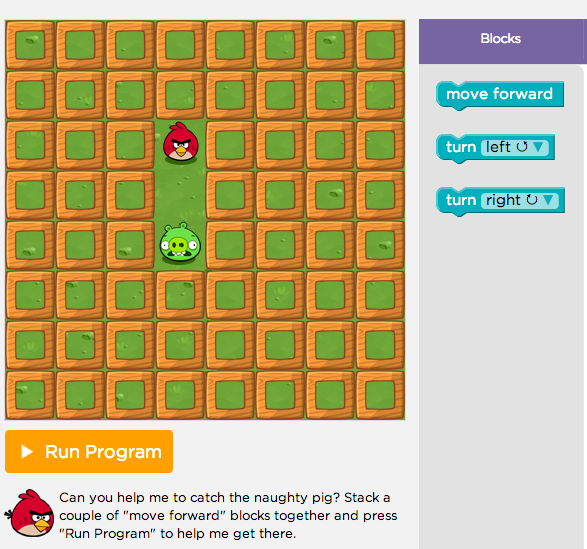# 1.2. Mazes Algorithms and Programs¶

## 1.2.1. Introduction and Goals¶

### Let's do some programming

MIT App Inventor is based on a blocks platform named Blockly. This demo is from Code.org's Hour of Code activity -- maybe you've seen it before? It will help you get a sense of the type of programming you will be doing in this course. It lets you write small Blockly programs (called scripts) to solve Maze puzzles. Can you solve all 20 problems?

#### Click on the maze to get started!## 1.2.2. Learning Activities¶

### Algorithms and Programs

As you learned in that activity, an Algorithm is a sequence of precise instructions that solves some problem or performs some computation. A program is an algorithm that is written in a programming language that runs on a computer and is often referred to as software. A program can be described by what it does and how the program statements accomplish its function. Each of the levels in the previous activity contained code segments which are collections of program statements that are part of a program.

The scripts you created in the Maze demo contain all the essential control structures that programmers use to design algorithms:

• Sequence. An algorithm is a sequence of precise statements (blocks).
• Selection (if/else). An algorithm can select between two alternative paths based on some condition.
• Repetition or Iteration (repeat). An algorithm can repeat a sequence of statements.

### Algorithms are Everywhere

Now that you've been introduced to the term algorithm and have an initial idea of what it means, you're going to discover algorithms everywhere. As you've grown up, you've learned all sorts of algorithms to solve everyday problems. For example, certainly we all know how to tie our shoelaces. But can you tie your shoelace in 2 seconds? You could if you follow this algorithm:

Here's an interesting fact: Computer scientists have proved that sequence, selection, and repetition are sufficient to build any algorithm that can be thought of. In other words, any algorithm can be expressed using only sequence, selection, and repetition.

### Food for Thought

Computer scientists write algorithms to solve problems. And we know now that sequence, selection, and repetition are sufficient to express any algorithm we can think of.

Are there algorithms we can't think of? Or, to put that another way, are there problems that can't be solved by an algorithm? What do you think?

That's one of the interesting questions we will take up in this course. In a few weeks, you'll know the answer.

## 1.2.3. Summary¶

In this lesson, you learned how to:

### Technical Terminology

Here is a table of the technical terms that were introduced in this lesson and that will be used throughout the course. You will see tables such as this in many of the lessons. If you hover over the term, its definition or description will pop up.
 algorithm control structure sequence selection repetition iteration